# Time + length - math problems

1. FighterA military fighter flies at an altitude of 10 km. From the ground position, it was aimed at an altitude angle of 23° and 12 seconds later at an altitude angle of 27°. Calculate the speed of the fighter in km/h.
2. Cyclist 12What is the average speed of a cycle traveling at 20 km in 60 minutes in km/h?
3. Two trainsThe train runs at speed v1 = 72 km/h. The passenger, sitting in the train, observed that a train long l = 75m in 3 s passed on the other track in the opposite direction. Calculate the speed of this train.
4. The swallow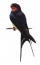The swallow will fly 2.8 km per minute. How many km will the swallow fly in one hour?
5. Self-oscillation period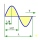The water in the vessel carried by the boy has a self-oscillation period of 0.8 s. What is the size of the boy's movement speed when the length of the boy's step is 60 cm? Give the result in m/s.
6. Two trains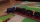Two trains departed from City A and City B against each other. They met after some time. The first train then took 9 hours to reach city B, and the second train took 4 hours to reach city A. In what proportion were the train speeds?
7. Doug bikedDoug biked 5 1/4 miles in 3/4 of an hour. What is his average speed?
8. Cheetah vs antelope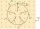When the cheetah began chasing the antelope, the distance between them was 120 meters. Although the antelope was running at 72km/h, the cheetah caught up with it in 12 seconds. What speed was the cheetah running?
9. Bike rideMarek rode a bike ride. In an hour, John followed him on the same route by car, at an average speed of 72 km/h, and in 20 minutes he drove him. Will he determine the length of the way that Marek took before John caught up with him, and at what speed did M
10. The touristThe tourist traveled 78km in 3 hours. Part of the journey went on foot at 6km/h, the rest of the trip by bus at 30km/h. How long did he walk?
11. Two citiesCities A and B are 200 km away. At 7 o'clock from city A, the car started at an average speed of 80 km/h, and from B at 45 min later the motorcycle is started at an average speed of 120 km/h. How long will they meet and at what distance from the point A i
12. Tourist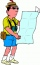A tourist walked an average speed of 3.5 km/h route in 6 hours. Calculate how many hours he would have passed at an average speed of 5.5 km/h.
13. Rocket start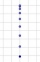The body launched vertically up returns to the start site in 6 seconds. What height did it have?
14. Athletic competitionIn a 400 meter athletic competition, a participant covers the distance as given below. find the average speed? first 80 meters 10 m/s next 240 meters 7.5 m/s last 80 meters 10 m/s
15. Velocipedes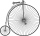In the 19th century, bicycles did not have a chain drive, and the pedals were connected directly to the wheel axis. This wheel diameter gradually increased until the so-called high bicycles (velocipedes) with a front wheel diameter of up to 1.5 meters, wh
16. Constant motionThe car has undergone constant motion 120 km in 1 hour 30 minutes. Determine its average speed. Determine how long car drove in the first 20 minutes and 50 minutes from the start of the move.
17. Wave parameters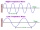Calculate the speed of a wave if the frequency is 336 Hz and the wavelength is 10 m.
18. GrandmotherMom walked out to visit her grandmother in a neighboring village 5km away and moved at a speed of 4km/h. An hour later, father drove down the same road at an average speed of 64km/h. 1) How long will take to catch mom die? 2) What is the approximate di
19. Two cyclists 2At the same time, two cyclists left the towns A and B at constant speeds. The first one going from town A to town B, and the second one from town B to town A. At one point of the trip they met. After they met, the first cyclist arrived at town B in 36min,
20. Two citiesThe car goes from city A to city B at an average speed of 70 km/h, back at an average speed of 50 km/h. If it goes to B and back at an average speed of 60 km/h, the whole ride would take 8 minutes less. What is the distance between cities A and B?

Do you have an interesting mathematical word problem that you can't solve it? Submit math problem, and we can try to solve it.

We will send a solution to your e-mail address. Solved examples are also published here. Please enter the e-mail correctly and check whether you don't have a full mailbox.

Please do not submit problems from current active competitions such as Mathematical Olympiad, correspondence seminars etc...

Do you want to convert length units? Do you want to convert time units like minutes to seconds?